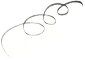# Easy Equation Solver

by Goran Nikolovski

## Easy Equation Solver is a software program designed to help users quickly and easily solve mathematical equations.

Operating system: Windows

Publisher: Goran Nikolovski

Antivirus check: passed

Last revision: Last week

Report a Problem

Easy Equation Solver is a revolutionary math solving software that makes solving equations and math problems easy and efficient. The software provides an intuitive user interface and powerful algorithms to quickly and accurately solve any equation.

Easy Equation Solver software provides a fast and efficient way to solve math equations.
Features:

• Solve any equation, no matter the complexity.

• Automatically recognize equations, terms and coefficients.

• User-friendly interface with easy-to-use controls.

• Step-by-step solution guide and graphs.

• Expand and simplify equations.

• Solve simultaneous equations.

• Solve equations with fractions and radicals.

• Solve equations with trigonometric functions.

• Support for all mathematics operations.

• Automatically generate equations.

• Generate and graph equations.

• Create customizable worksheets.

• Multiple options for equation solving.

• Comprehensive help and support.

Easy Equation Solver is the perfect tool for students and professionals alike who need to solve math problems quickly and accurately. The software is designed to make it easy to input equations, terms and coefficients, and provides a step-by-step guide of the solution. The software can also generate equations, expand and simplify equations, and graph equations. Additionally, the software can solve simultaneous equations, equations with fractions and radicals, and equations with trigonometric functions.

The software is intuitive, easy-to-use and feature-rich, making it the perfect choice for anyone who needs to solve equations quickly and accurately. It also includes a comprehensive help and support system, so if you ever need help with using the software, you can quickly and easily get assistance.

Easy Equation Solver is the perfect tool for anyone who needs to solve math problems quickly and accurately. With its intuitive user-interface, powerful algorithms and comprehensive help and support system, it is the perfect choice for anyone who needs to solve equations.
This software shall be compatable with Windows or Mac OSX Operating Systems and shall provide an easy to use equation solver application. The software shall provide a user interface that is easy to use and intiutive. The software shall allow the user to enter an equation and recieve a solved result. The software shall also provide the ability to store equations for later use. The software shall also have an in-built help section to provide assistance with using the software.
Charlie O.

This sofware is great for solving many math equations and with just few clicks, I was able to get the anwser to my problem easily.
Kayden Kupcho

This software provides a great tool for solving equations quickly and accurately.
Joseph N*******f

This software is a tool designed to solve mathematical equations with ease. It supports a wide range of equations including linear, quadratic, and polynomial equations. The software provides step-by-step solutions and allows users to input complex equations using a user-friendly interface. It also includes a graphing feature to visualize equations and their solutions. The software is compatible with Windows and Mac operating systems.
Noah R.

Easy Equation Solver software is a user-friendly tool for solving mathematical equations.